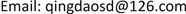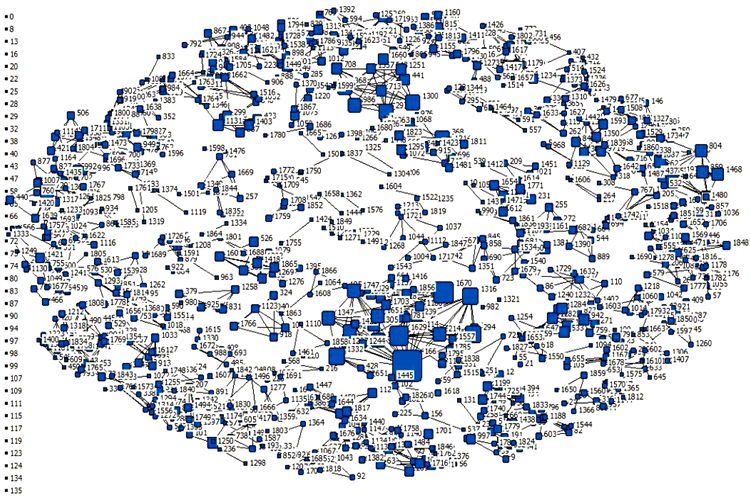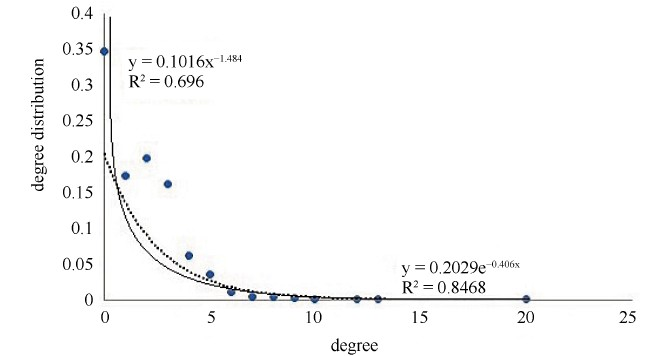﻿ 基于社交网络的论文合著情况分析——以偏微分方程领域的相关论文为例 Analysis of the Co-Authorship of Papers Based on Social Networks—Taking the Relevant Papers in the Field of Partial Differential Equations as an Example

Hans Journal of Data Mining
Vol. 09  No. 02 ( 2019 ), Article ID: 29511 , 7 pages
10.12677/HJDM.2019.92004

Analysis of the Co-Authorship of Papers Based on Social Networks

—Taking the Relevant Papers in the Field of Partial Differential Equations as an Example

De Sun

Shandong University of Science and Technology, Qingdao ShandongReceived: Mar. 8th, 2019; accepted: Mar. 22nd, 2019; published: Mar. 29th, 2019ABSTRACT

This paper combines the relevant data of the papers in the field of partial differential equations in the last five years of CNKI, establishes a network of papers, analyzes the network characteristics of the papers, and puts forward some problems in the co-authoring of the papers and the solutions. Firstly, this paper reflects the characteristics and laws of the co-existing phenomenon of the papers in the field of partial differential equations from the perspective of the co-authorship rate and the number of authors. Then, UCINET software is used to construct the paper co-authoring network and analyze the small world characteristics of the network and no scale characteristics. Finally, the problems and development countermeasures of the co-authorization of papers related to the field of partial differential equations in the past five years are proposed.

Keywords:Paper Co-Authoring Network, Field of Partial Differential Equations, Network Characteristics, Paper Co-Authorship

——以偏微分方程领域的相关论文为例Copyright © 2019 by author(s) and Hans Publishers Inc.1. 引言

2. 偏微分方程领域论文的合著情况研究

3. 论文合著情况分析

1) 论文合著率研究

CNKI中偏微分方程领域论文各年的合著率如表2所示。Table 2. Cohesion rate of papers in the field of partial differential equations

2) 论文著者数研究Table 3. The number of different authors in the paper

4. 偏微分方程领域论文的合著网络及其分析

4.1. 论文合著网络的建立

${a}_{ij}=\left\{\begin{array}{l}1,第i个作者和第j个作者合作过论文\hfill \\ 0,第i个作者和第j个作者没有合作过论文\hfill \end{array}$Figure 1. Co-authored network of papers in the field of partial differential equations

4.2. 网络特征属性的描述

1) 最短路径

$\begin{array}{c}\text{L}=\frac{1}{\frac{1}{2}N\left(N-1\right)}\underset{i>j}{\sum }{d}_{ij},i,j=1,\cdots ,N.\end{array}$ (1)

2) 聚类系数

$\begin{array}{c}{C}_{i}=\frac{n}{{C}_{k}^{2}}=\frac{2n}{k\left(k-1\right)},i=1,2,\cdots ,N.\end{array}$ (2)

3) 度与度分布

4.3. 论文合著网络的网络特征分析

1) 小世界性

2) 无标度性Figure 2. Degree distribution map of paper co-authorship network

5. 结论及建议

5.1. 结论

1) 2014~2018年5年期间偏微分方程领域的论文合著率总体上呈上升趋势，但总体而言尚处于较低水平，且上升速率比较缓慢，这说明偏微分方程领域的论文合著情况尚不完善，还存在很大的发展空间。

2) 在偏微分方程领域的论文中，独著论文所占比例最高，且大部分合著论文仅限于两人到三人合著，这说明偏微分方程领域相关论文的合著度较低，尚未形成更大的合著网络。

3) 从构建的偏微分方程领域论文合著网络来看，网络中有很多节点的度为0，也就是说，存在相当数量的作者没有与其他作者合作过；而有一些节点的度大于9，有很多合作过的作者，这说明不同作者之间的论文合著情况有较大差异。

4) 该论文合著网络具有无标度特性，就是说网络中大部分作者具有较少的合作，而只有一小部分作者有很多合作，且作者合著主要以2~3人合著为主，合著规模比较小。

5.2. 建议

Analysis of the Co-Authorship of Papers Based on Social Networks—Taking the Relevant Papers in the Field of Partial Differential Equations as an Example[J]. 数据挖掘, 2019, 09(02): 27-33. https://doi.org/10.12677/HJDM.2019.92004

1. 1. 孟繁华. 基于复杂网络的供应链建模及其鲁棒性分析[D]: [硕士学位论文]. 北京: 华北电力大学, 2018.

2. 2. 世攀科, 徐锡, 程文聪. 大规模网络拓扑属性特征及模型分析[C]//中国计算机学会. 中国计算机大会论文集: 2008年卷. 西安: 中国计算机学会, 2008: 464-472.

3. 3. 刘贵香, 蔡永明, 刘璐, 英玉超. 社会网络信息传播机制研究——以新浪微博为例[J]. 经贸实践, 2018(6X): 69-70.

4. 4. 施梅, 胡婷婷. 我国科技期刊论文合著现象分析——以图书情报学核心期刊为例[J]. 出版发行研究, 2018.